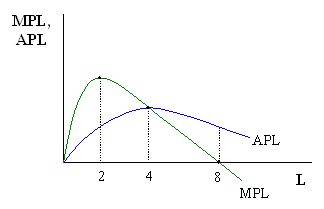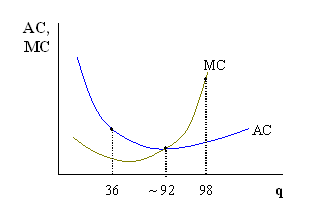Barry Haworth
University of Louisville
Department of Economics
Economics 201

### Product and Cost Curves

The point of this handout is to illustrate some basic points about product and cost curves. Below, we will address various ways of measuring output and learning how factors contribute to producing that output. When we measure the cost of production, we'll want to make some similar calculations there.

Product Curves
Consider the table below. The table assumes that a certain good is produced by using labor and capital (K), where varying amounts of labor are hired to work with a fixed amount of capital (4 units). For the sake of this example, let's assume that these 4 units of capital are 4 pieces of equipment (e.g. 4 machines).

Each combination of labor (L) and capital (K) produces a different level of output (Q). Eventually, however, adding laborers adds nothing to output and will even actually decrease output if more laborers were hired. Once we know the output, it is possible to determine how our variable factor contributes to the production of that output. To do so, we calculate the average product of labor (APL) and marginal product of labor (MPL) by computing Q/L and DQ/DL respectively.

 L K Q APL MPL 0 4 0 - - 1 4 10 10 10 2 4 36 18 26 3 4 60 20 24 4 4 80 20 20 5 4 90 18 10 6 4 96 16 6 7 4 98 14 2 8 4 98 12.2 0 9 4 90 10 -8

If we take this information and insert it into a graph, we have:The graph shows several points where the relative position of the average and marginal product curves tell us something about how the average product of labor is changing. This illustrates the average-marginal rule where when a marginal value is less than (greater than) an average value, the average is falling (rising). When the two are equal, the average is constant - which implies that the average should be at a maximum or minimum point. On the graph, we see that when 4 units of labor are hired, MPL = APL and APL is at a maximum. When 2 units (8 units) are hired, APL < MPL and APL is rising (APL > MPL and APL is falling).

Cost Curves
Now, suppose we assume that this firm must pay \$20 for each unit of labor hired, and \$25 for each unit of capital purchased. This information allows us to take the data from the table above and measure (economic) costs. The total cost of the variable factor and total cost of the fixed factor (i.e. Total Variable Cost, TVC, and Total Fixed Cost, TFC) add up to the (overall) Total Cost, TC.

Just as we calculated average and marginal product above, we can calculate the average (total) cost and marginal cost here. Average Cost, AC, is calculated by dividing total cost by output (i.e. TC/Q). Marginal Cost, MC, is calculated as the change in total cost caused by a change in output (i.e. DTC/DQ).

 L K Q TVC TFC TC AC MC 0 4 0 0 100 100 - - 1 4 10 20 100 120 12.00 2.00 2 4 36 40 100 140 3.89 0.77 3 4 60 60 100 160 2.67 0.83 4 4 80 80 100 180 2.25 1.00 5 4 90 100 100 200 2.22 2.00 6 4 96 120 100 220 2.29 3.33 7 4 98 140 100 240 2.45 10.00 8 4 98 160 100 260 2.65 - 9 4 90 180 100 280 3.11 -

As above, we can take the information from the table and draw the graph of average and marginal cost:Just as above, we see the average-marginal rule at work when comparing the AC and MC curve at 36 and 98 units of output. When AC = MC, at about 92 units of output, AC is at a minimum point.